# Paper Walkthrough — Matrix Calculus for Deep Learning (Part 1 / 2)

1. The foundations of Neural Networks, such as what are neurons, what are weights and biases, ReLU etc.(reference).
2. Basic differentiation and the concept of partial derivatives.

# Affine Transformation

Think about the equation for a straight line, with the independent variable y and the dependent variable x: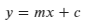Eq.1 : Straight Line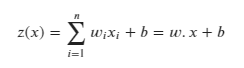Eq.2 : Output of a single neuron in a neural network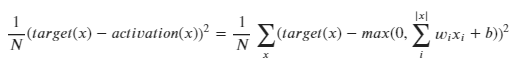Eq.3 : Comparison of the predicted and target values

The gradient of a function is simply the matrix of its partial derivatives.

# Jacobian

A Jacobian is nothing but a stack of gradients. Overly simply, we can just place gradients on top of each other to get the jacobian. Say we have a vector function, which is in turn made up of two scalar functions f(x, y) and g(x, y):

# Generalization of the Jacobian

Consider a column vector x, of size n, i.e. |x| = n.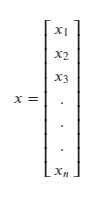Eq.9 : Column vector x of size n.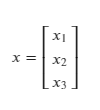Eq.10 : Column vector of size 3.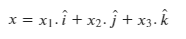Eq.11 : How vector x would be introduced in Physics in the subcontinent

# Generalization ramp-up

Say we have a vector y, such that y = f(x), where:

1. x is a vector of size n.
2. y is a vector of m scalar valued functions.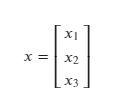Eq.12 : Column vector x, n = 3 (size)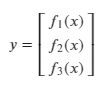Eq.13 : Column vector y, m = 3 (size)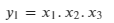Eq.14 : y₁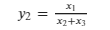Eq.15 : y₂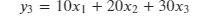Eq.16 : y₃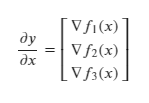Eq.17 : Jacobian of y.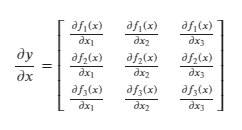Eq.18 : Jacobian of y, with the gradients expanded.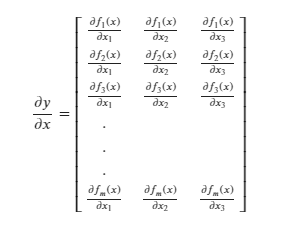Eq.19 : A more general Jacobian, where y has a size of m and x has a size of 3.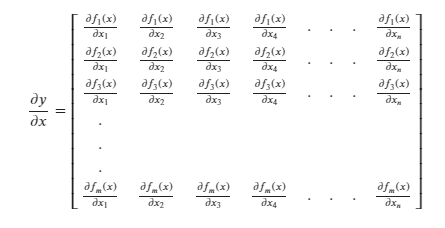Eq.20 : A more general Jacobian, where y has a size of m and x has a size of n.

# Jacobian — Analyzing a few cases

For all cases, y = f.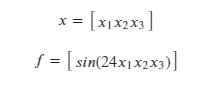Eq.21 : f and x are 1 x 1 scalars.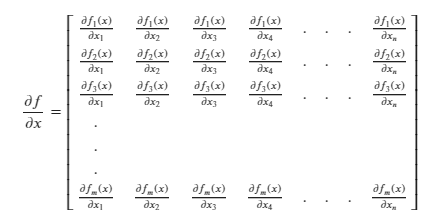Eq.22 : Generalized Jacobian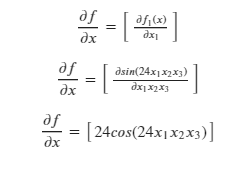Eq.23 : Jacobian of a scalar with respect to a scalar.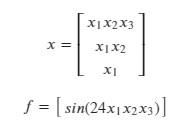Eq.24 : f is a 1x1 scalar and x is a 3x1 vector.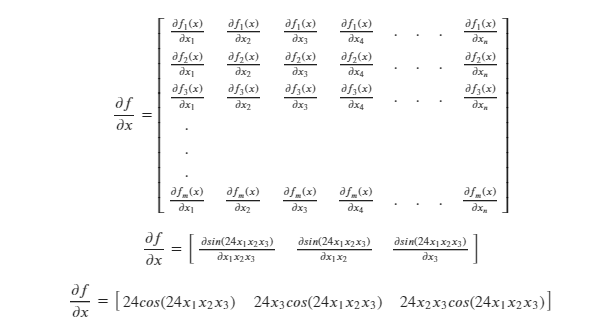Eq.25 : Jacobian of a scalar with respect to a vector.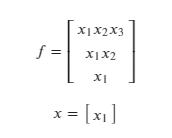Eq.26 : f is a 3x1 vector and x is a 1x1 vector.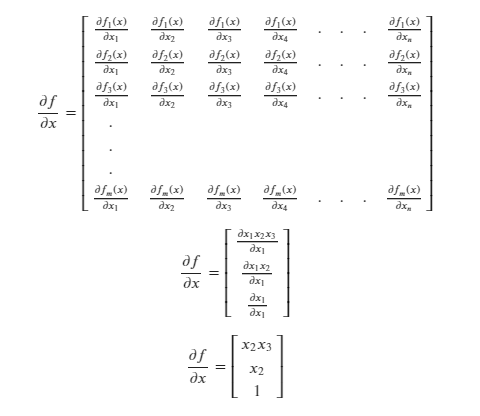Eq.27: Jacobian of a vector with respect to a scalar.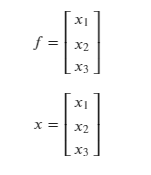Eq.28 : f is a 3x1 vector and x is a 3x1 vector.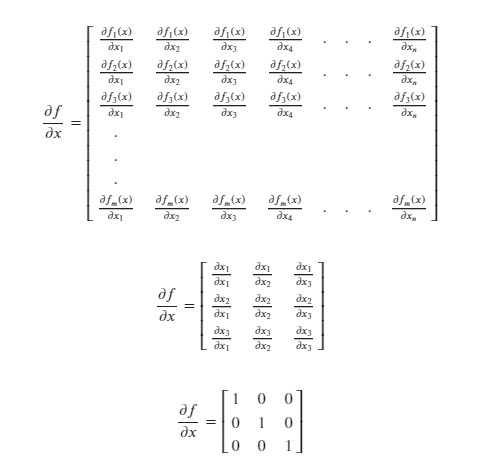Eq.29: Jacobian of a vector with respect to a vector.

# Summary

To sum up all of the cases mentioned above: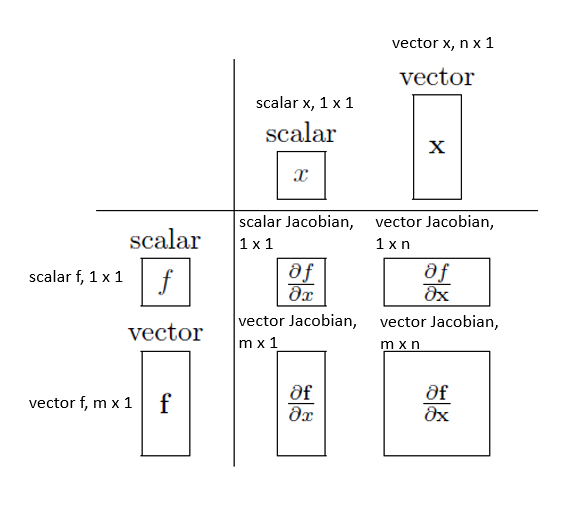Eq.30 : Variation of Jacobian sizes with input.

--

--## Kaushik Moudgalya

Computer Science Master’s student at the University of Montreal, specializing in Machine Learning.